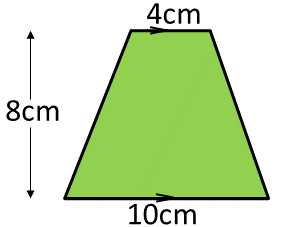# C Program Area Of Trapezium – 3 Ways | C Programs

C Program to find the area of a trapezium. In this particular article, we will brief in on the different ways the area of a trapezium is calculated in C programming. The methods discussed here are as follows:

• Using Standard Method
• Using Function
• Using Pointers
• Using Macros

A Trapezium is a quadrilateral which has a single pair of parallel opposite sides. A square, rhombus and a rectangle can be considered as trapeziums since these have at least one pair of parallel opposite sides.

The area of a trapezium after simple derivation can be considered to be

= 1/2 * (sum of the length of the parallel sides) * height.

A trapezium is given in the following image:As you can see, the area of the trapezium shown in the image can be easily calculated. The area is:

1/2 * (4 + 10) * 8 = 56 sq. units.

## Using Standard Method

1)For the area of a trapezium, we need the values of base1,base2, height.

2)By substituting the values of base1,base2,height into the formula area=((base1+base2)/2)*height,we will get area of trapezium,the value will store into the variable “area”.

output:

### Using Function

1)We are using float area(float a, float b, float h) function to calculate the area of the trapezium.

2)We are calling the function using area(a,b,h), then called function will calculate the area of the trapezium and return the value.

3)The return value will be assigned to the variable “A”.

output:

#### Using Pointers

1)We are passing the addresses as arguments in the calling function AOT(&b1,&b2,&h,&area).

2)The called function AOT(float *a,float *b,float *h,float *area) calculate the area by retrieving the values at that addresses.

3)The calculated value will store into the pointer variable “area”.

output:

##### Using Macros

1)area(b1,b2,h) is symbolic name to the expression ((b1+b2)/2)*h

2)area(b1,b2,h)replaced with that expression given at #define.

output:
x

## C Program To Print Number Of Days In A Month | Java Tutoring

C program to input the month number and print the number of days in that ...